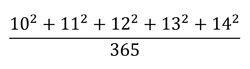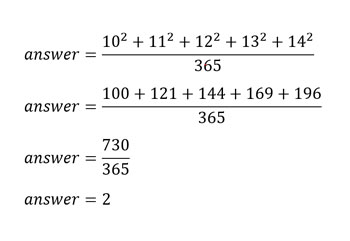# What's new## A past puzzle

By David, 26 August 2020 Brainteaser

Here’s a beautiful artwork from 1895, titled Mental calculation. In public school of S. A. Rachinsky, painted by Nikolay Bogdanov-Belsky.

Can you solve the puzzle the kids are pondering?

If you can’t quite see the equation, here it is:Scroll down or click for a hint, or the answer!## Brainteaser hint

• Although the kids in the painting are trying to do it in their heads, you can always use a pencil!
• Remember that teachers often like questions with nice round answers.
• 102 means 10 x 10. Calculate these first!Here’s the working:For more brainteasers and puzzles for kids, subscribe to Double Helix magazine!Categories:

## Similar posts

1.For doing mental arithmetic it’s handy to know a few arithmetic shortcuts.
This problem is easier to do in your head if you know that (x + y)(x+y) = xx + 2xy + yy
If you set x=10 and y to 0, 1, 2, 3, 4
then you can write the sum 10×10 + 11×11 + 12×12 + 13×13 + 14×14
as
10×10 + (10+1)x(10+1) + (10+2)x(10+2) + (10+3)x(10+3) + (10+4)x(10+4) =
100
+ 100 + 20 + 1
+ 100 + 40 + 4
+ 100 + 60 + 9
+ 100 + 80 + 16

= 5×100 + 20+40+60+80 + 1+4+9+16
= 500 + 200 + 30
=730

I can do that in my head much more easily than I can calculate squares and add up three-digit numbers easily.